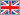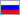Home   Back

# IEEE754

## The program IEEE754 - format converter numbers IEEE754 c absolute accuracy of reporting results.

The registration is free

### §1 Brief description

The program is designed to convert real numbers into a format single or double precision IEEE754 standard in both directions. The program displays an absolutely accurate value of decimal or binary numbers presented in the format of IEEE754. The initial number and the conversion results can be represented in exponential form.

System requirements:
• unlimited
• OS W2k/XP/W7

### §2 Program Description IEEE754 v.1.0

IEEE754 program allows you to:
• enter the original decimal digits until 1074 in a simple or exponential form;
• convert the original number in the format of the single and double precision IEEE754;
• calculate the difference between the original and the number of the same date in the format presented IEEE754;
• calculate the difference between the ones of single and double precision;
• compute the exact value of decimal numbers presented for IEEE754 format;
• compute the exact value of the binary number for the numbers presented in the format IEEE754;

Fig. 1. Window IEEE754 v.1.0The program does not use a mathematical possibility of a microprocessor.
Since the microprocessor is not able to produce as accurate calculations.
The program simulates the mathematical calculations performed by hand on paper.
The variables in the program are presented in the form of lines of ASCII characters.
Type of variables presented in the form of a real fixed-point numbers.
The maximum length of an entire part of the 1074 level.
The maximum length of 1074 bit fractional part.
All mathematical operations are performed with this data type.
Calculation speed is low, as all mathematical operations are performed as operations on text strings.

The program is designed for the analysis of errors in planning for computer calculations.

Back   Home